Next: Standing Waves: Preserving Isotropy Up: Initial Conditions on the Previous: Initial Conditions for Field

## Initial Conditions for Field Derivative Fluctuations

To calculate the field derivatives it is necessary to know the time dependence of the vacuum fluctuations being considered. The full time dependence comes from several sources. First, there is an oscillatory term. We discuss in section 6.3.4 below the use of the plus or minus sign in this term. Next, all the modes have an extra factor ofrelative to their Minkowski space values. This can be seen for example from equation (6.55). This extra factor ensures that the physically meaningful quantity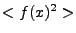depends on the physical rather than the comoving momenta of the modes in the box. There is an additional time dependence that arises from the fact that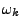is time dependent, both because of the scale factor multiplying the mass term and because of possible time dependence of the effective mass itself. Finally there is the issue of converting to program variables, which can involve rescaling the field values and time coordinates in a time dependent way. Here we calculate the field derivatives taking all of these effects into account except the time dependence of. Section 6.3.7 explains the justification for this approximation.

Using this approximation the full time dependence of the modeis given by the square root of the rms amplitude (equation (6.62)) times, i.e.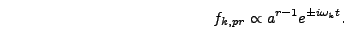(6.70)

Denoting derivatives with respect to the program timewith primes we have(6.71)

Taking the initial scale factor to be one this becomes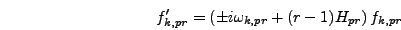(6.72)

where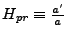. The calculation of the initial value of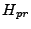is described in section 6.3.6.Next: Standing Waves: Preserving Isotropy Up: Initial Conditions on the Previous: Initial Conditions for Field